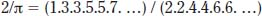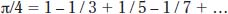NextPrevious

# How was the value of pi determined arithmetically?

One of the earliest mathematical formulas for pi was determined by English mathematician John Wallis (1616–1703), who wrote the notation as:Another more commonly-recognized notation for pi is often attributed to German philosopher and mathematician Baron Gottfried Wilhelm Leibniz (1646–1716), but is more likely the work of Scottish mathematician James Gregory (1638–1675):Both are amazing examples of something that was not only figured out using geometric methods, but also arithmetic methods.

Close

This is a web preview of the "The Handy Math Answer Book" app. Many features only work on your mobile device. If you like what you see, we hope you will consider buying. Get the App